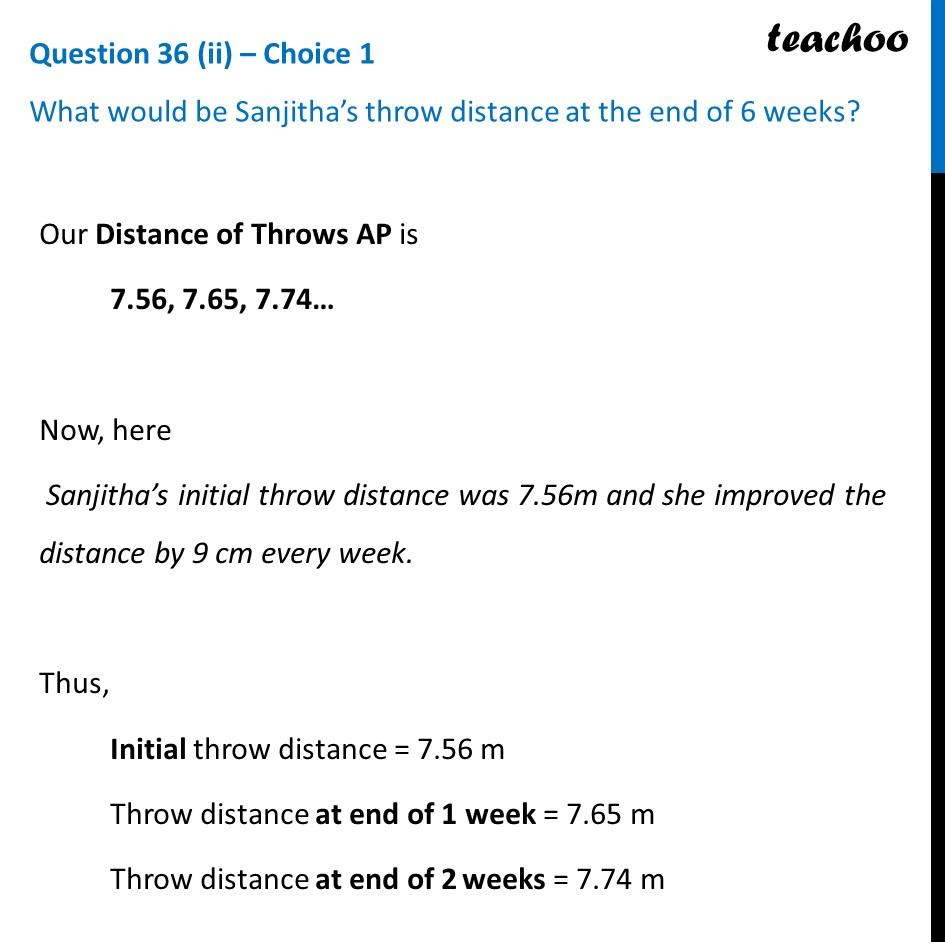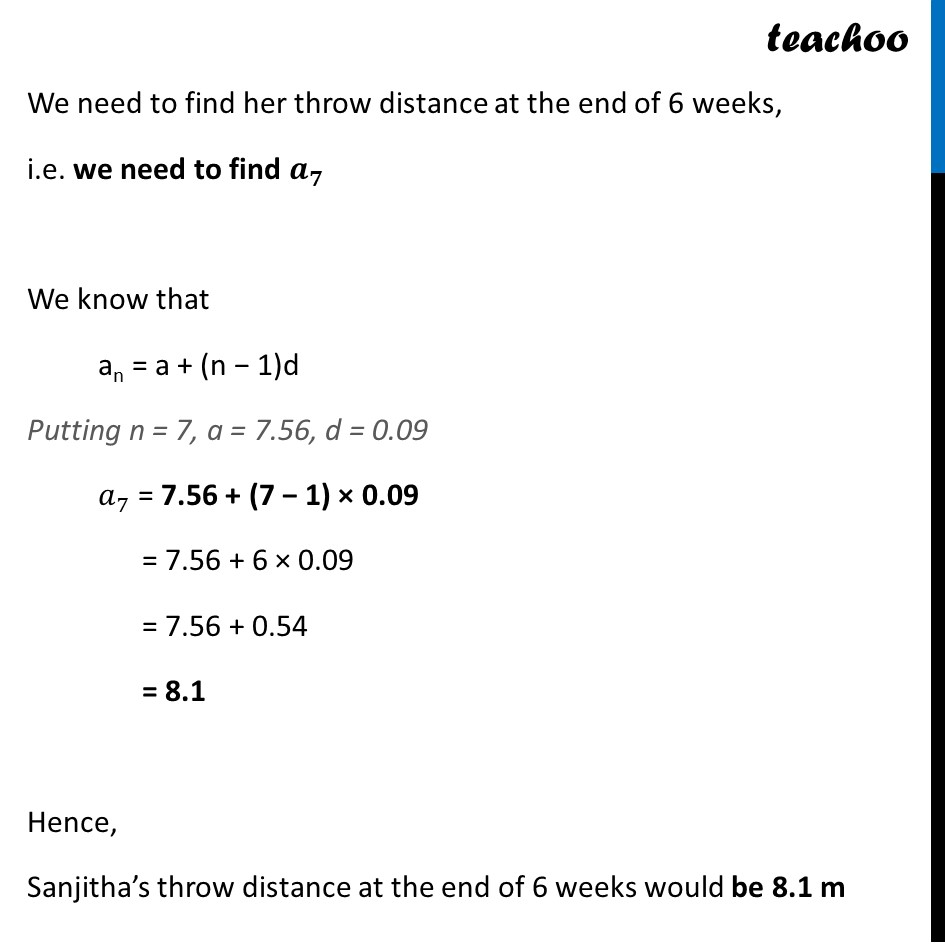CBSE Class 10 Sample Paper for 2024 Boards - Maths Standard

Class 10
Solutions of Sample Papers for Class 10 Boards

## What would be Sanjitha’s throw distance at the end of 6 weeks?Learn in your speed, with individual attention - Teachoo Maths 1-on-1 Class

### Transcript

Our Distance of Throws AP is 7.56, 7.65, 7.74… Now, here Sanjitha’s initial throw distance was 7.56m and she improved the distance by 9 cm every week. Thus, Initial throw distance = 7.56 m Throw distance at end of 1 week = 7.65 m Throw distance at end of 2 weeks = 7.74 m We need to find her throw distance at the end of 6 weeks, i.e. we need to find 𝒂_𝟕 We know that an = a + (n − 1)d Putting n = 7, a = 7.56, d = 0.09 𝑎_7 = 7.56 + (7 − 1) × 0.09 = 7.56 + 6 × 0.09 = 7.56 + 0.54 = 8.1 Hence, Sanjitha’s throw distance at the end of 6 weeks would be 8.1 m